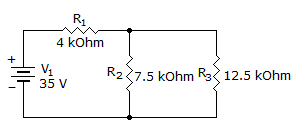# Electronics - Series-Parallel Circuits - Discussion

### Discussion :: Series-Parallel Circuits - General Questions (Q.No.12)

12.

What are the branch currents I2 and I3?[A]. I2 = 4 mA, I3 = 2 mA [B]. I2 = 4.5 mA, I3 = 2.5 mA [C]. I2 = 2.5 mA, I3 = 1.5 mA [D]. I2 = 5.5 mA, I3 = 3.5 mA

Explanation:

No answer description available for this question.

 Madhu said: (Jul 23, 2011) I2 is 9.56mA

 Mike B said: (Sep 18, 2011) Total current I(1): R(2) and R(3) are together 4k7 This in series with R(1) is 8k7 I(1) = 35/8k7 = 4mA I(2) = 4*12.5/(12.5+7.5) = 2.5mA I(3) = 4mA - 2.5mA = 1.5mA Or am I doing something wrong??

 Visakh said: (Oct 4, 2011) Anyway the total current drawn from the source is 35/(4+4.69) ie 4.029mA, tat means always I2 or I3 may be less than or equal to 4.029mA

 Prof. X said: (Apr 17, 2012) R2 & R3 = 4687.5 ohm's plus R1 = 8687.5 ohms It = 4ma so I2 = 2.5ma I3 = 1.5ma

 Carlo Gonzales said: (Jul 11, 2013) I2 = 7/2780 A. I3 = 21/13900 A. Why has it been multiplied by 2?

 Mukesh said: (Jul 20, 2013) Total resistance of the circuit is: 4K+(12.5K||7.5K) = 4K+4.6875k) = 8.6875K. Total current = 35/8.6875K = 4.028776 mA. I2 = 12.5K*4.028776 mA/(20K) = 2.517985 mA. I3 = 7.5K*4.028776 mA/(20K) = 1.510791 mA. Hence none of the options above are correct.

 Junayd said: (Mar 31, 2014) Using CDR. Req(parallel) = 4.65. So I2 = 4.65/7.5 X 4.02mA = 2.49mA. I3 = Itotal-I2 = 4.02-2.5 = 1.5mA.

 Blueknight802 said: (Jan 30, 2016) Nodal Analysis: (V-35)4 + (V-0)7.5 + (V-0)12.5 = 0. V = 18.84 V. I2 = V/7.5 = 2.518 mA. I2 = V/12.5 = 1.511 mA.

 Bhargavi said: (Jan 12, 2019) Why we are taking v2 and v3 as zeros?